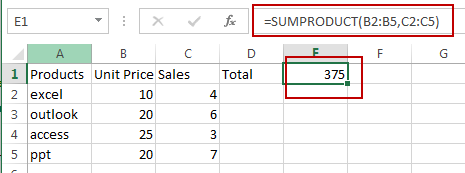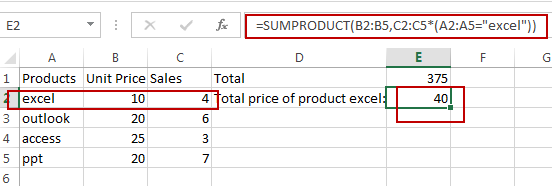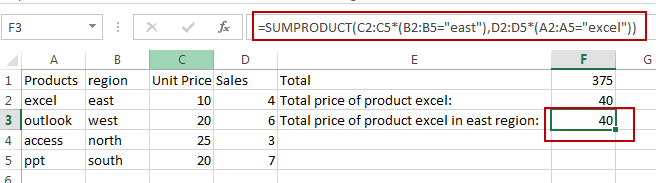# How to Multiply two columns and Sum The Result in Excel

This post will guide you how to multiply the values in two columns and then sum the result of all rows in Excel. How do I multiply two columns and add up the results using SUMPRODUCT function in Excel. How to Use the Excel SUMPRODUCT function with one or more criteria in Excel.

## Multiply Two Columns And Sum the Result

Assuming that you have a list of data in range A1:C5 in which contain sales data and unit price data. And you want to multiply those two columns and then sum the result in Excel. You can use a formula based on the SUMPRODUCT function to achieve the result. Like this:

`=SUMPRODUCT(B2:B5,C2:C5)`

Type this formula into a black cell to place the last sum result, and press Enter key to apply this formula.## Multiply Two Columns And Sum the Result with One Criteria

If you want to multiply those two columns and sum the result based on the certain product “excel”, you can also use the SUMPRODUCT function to create a formula. Like this:

`=SUMPRODUCT(B2:B5,C2:C5*(A2:A5="excel"))`

Type this formula into a blank cell to place the total price of product “excel”, and press Enter key to apply this formula.## Multiply Two Columns And Sum the Result with Two or More Criteria

If you want to multiply two columns and sum the result to get the total price of product “excel” in the east region. How to calculate it. You can use a formula based on the SUMPRODUCT function to achieve the result. Like this:

`=SUMPRODUCT(C2:C5*(B2:B5="east"),D2:D5*(A2:A5="excel"))`

Type this formula into a blank cell and press Enter key to apply this formula to get the total price of product excel in east region.### Related Functions

• Excel SUMPRODUCT function
The Excel SUMPRODUCT function multiplies corresponding components in the given one or more arrays or ranges, and returns the sum of those products.The syntax of the SUMPRODUCT function is as below:= SUMPRODUCT (array1,[array2],…)…